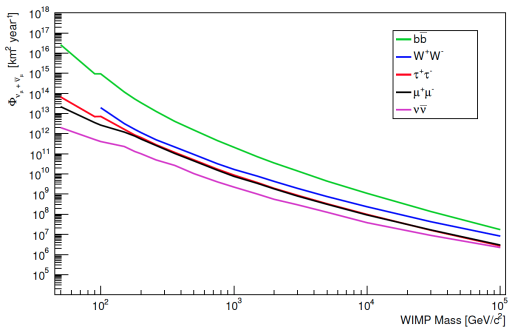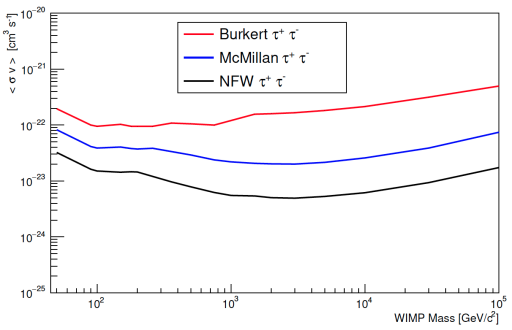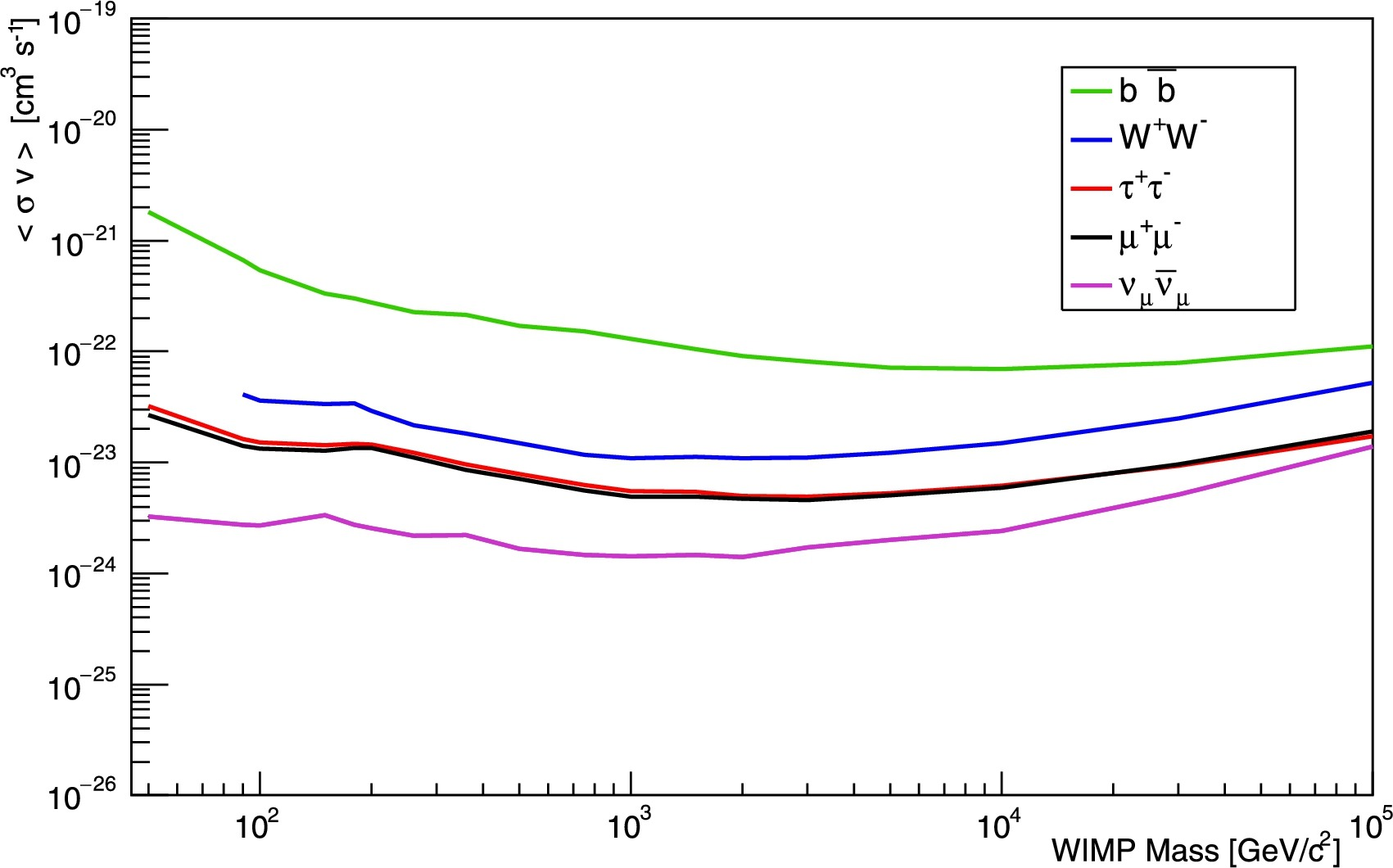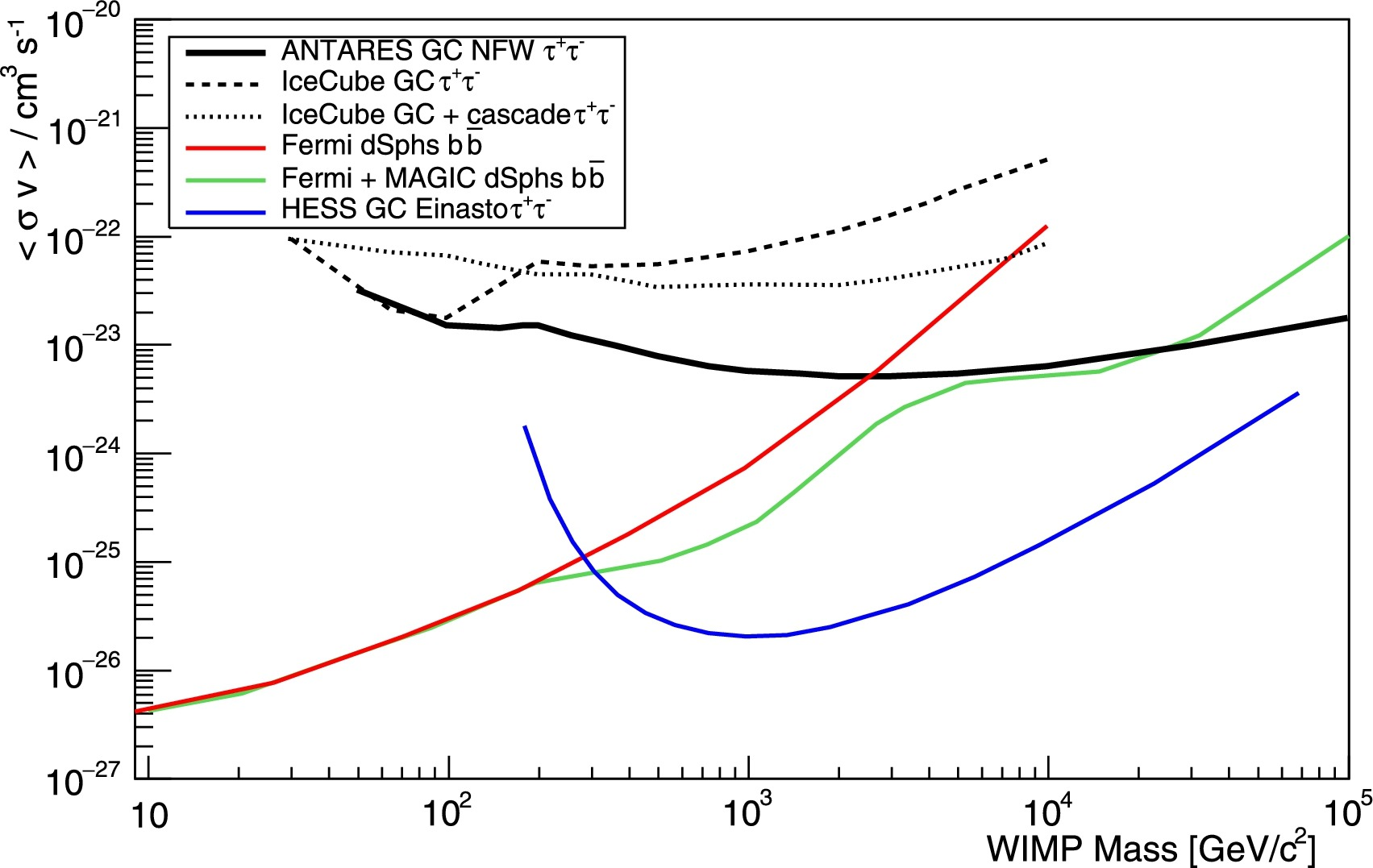# ANTARES search on Dark Matter in the Milky Way (Erratum)

The ANTARES Collaboration has submitted an erratum to the paper “Results from the search for dark matter in the Milky Way with 9 years of data of the ANTARES neutrino telescope” [Phys. Lett. B 769 (2017) 249-254]. In the paper, limits on the neutrino flux for a given mass MWIMP and annihilation channel were calculated with a quantity called the “integrated acceptance” (see Eq. 8, page 252 of the paper). This is defined as the convolution of the energy-dependent detector effective area with the neutrino spectrum at the detector’s location, derived from WIMPs annihilation in each selected channel. The neutrino spectra were computed using the tabulated version of PPPC4. To produce the convolution over the neutrino energy spectrum, a too coarse numerical approximation was defined to fold the pre-binned tabulated values into our effective area. This led to an overestimation of the integrated acceptance whose impact is proportional to the maximum neutrino energy considered, hence to the WIMP mass. The effect had consequences on Figs. 3 to 6 of the original paper.
In the Erratum, the same PPPC4 model was used but the convolutions over the neutrino energy have been produced analytically using the neutrino spectra obtained from the Mathematica functions reported in the website. As a result, the 90% C.L limits reported in Figs. 3 to 6 are weaker for WIMP masses larger than 1 TeV/c2. An erratum showing these results has been accepted for publication by Phys. Lett. B. containing the new plots, that can be seen below.90% C.L. upper limits on the neutrino flux from WIMP annihilations in the Milky Way as a function of the WIMP masses for the different channels considered. For this plot the NFW profile was used.90% C.L. limits on the thermally averaged annihilation cross–section, <σv>, as a function of the WIMP mass for the three considered halo models for the τ+τ- channel.90% C.L. limits on the thermally averaged annihilation cross-section, <σv>, as a function of the WIMP mass for all annihilation channels using the NFW halo profile.90% C.L. limits on the thermally averaged annihilation cross–section, <σv>, as a function of the WIMP mass in comparison to the limits from other experiment. The results from IceCube and ANTARES were obtained with the NFW profile.